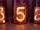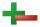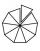#### Number of examples found: 103

• Evaluate expression 2Evaluate expression with negatives: (-3)+4+(-8)+(-6)+4+(-1)3 3/4 + 2 3/5 + 5 1/2 Show your solution.
• Length subtractingExpress in mm: 5 3/10 cm - 2/5 mm
• Product of the sum and differenceCalculate the product of the sum and difference of numbers -7 and -2.
• Evaluate expressionEvaluate expression using BODMAS rule: 1 1/4+1 1/5÷3/5-5/8What will be hexadecimal number 303 as decimal number?
• NoontimeIn the morning temperature was 110°F. By noontime it has gone up by 15°F. What was the noon temperature. (Give your answer in °F)
• Roses and tulipsAt the florist are 50 tulips and 5 times less roses. How many flowers are in flower shop?Add this two mixed numbers: 1 5/6 + 2 2/11=
• Expression 6Evaluate expression: -6-2(4-8)-9
• Opposite numbersCalculate opposite numbers (additive inverse) to given ones:
• Evaluate expressionIf x=2, y=-5 and z=3 what is the value of x-2y
• Simplify 3Simplify mixed numerals expression: 8 1/4- 3 2/5 - (2 1/3 - 1/4) Show your solution.
• This morningThis morning it was 80 degrees F outside the temperature increased 12 degrees F. What is the temperature now?
• Expression 1What is 7+8-(5×2)+5-4+(6×(5-3)+6)-(8+10)-7+6?
• Sum of fractionsWhat is the sum of 2/3+3/5?
• I think numberI think number.When I add 841 to it and subtract 157, I get a number that is 22 greater than 996. What number I thinking?
• Mixing Celsius and FahrenheitAdd up three temperatures: 5°F +6°F +0°CWhy does 1 3/4 + 2 9/10 equal 4.65? How do you solve this?Sum of interiol angles of 9-gon is: